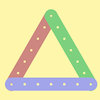#### You may also like### I'm Eight

Find a great variety of ways of asking questions which make 8.### Let's Investigate Triangles

Vincent and Tara are making triangles with the class construction set. They have a pile of strips of different lengths. How many different triangles can they make?### Noah

Noah saw 12 legs walk by into the Ark. How many creatures did he see?

# How Odd

##### Age 5 to 7Challenge Level

We did not receive any satisfactory submissions this time, so here are some from past years.

Many of you were careful to say that you only counted numbers BETWEEN the two numbers given, so you didn't include the numbers themselves.  That's important to get clear, I think.

Matthew and Luca from Dunchurch Boughton Junior School described what they noticed:

If the two numbers are consecutive (I think here they mean the starting numbers of each set of numbers) and the last numbers are the same, the answer will always be exactly the same.

Here are some examples:
1-9: 3, 5, 7. = three odds
2-9; 3, 5, 7. =  three odds

3-15: 5, 7, 9, 11, 13. = five odds
4-15: 5, 7, 9, 11, 13. = five odds

123-131: 125, 127, 129. = three odds
124-131: 125, 127, 129. = three odds

Moana from the Canadian Academy explained what Matthew and Luca noticed by saying:

We figured out that after an odd number, it's an even number and since we don't count even numbers it doesn't change.  (I think by 'it' in the second sentence Moana means the number of odd numbers.)

James from Edenlode wrote:

3 odd numbers between 3-11!
3 odd numbers between 4-11!!!
The same number of odd numbers in between!!!!!
Because the next odd number after 3 is 5!!!!!!!

Some numbers with 3 odds in between are 0 and 6 or 0 and 7!
Some numbers with 4 odds in between are 0 and 8 or 0 and 9!!!
Some numbers with 5 odds in betwen are 0 and 10 or 0 and 11!!!!!
Some numbers with 6 odds in between are 0 and 12 or 0 and 13!!!!!!!

If I was explaining to someone else how to work out how many odds between two numbers I would say 'If you start on an even number and land on an even number then the number of odds is half the number you count on. If you start on an even number and land on an odd number, then the number of odds is half the number you count on, plus one more'.

I love maths.

I wonder what happens when you start on an odd number and land on an even number?  Or if you start on an odd number and land on an odd number?  James also asked "Do you notice anything about my exclamation marks?".

Perhaps some of you thought about it in a slightly different way?  Let us know if you did!